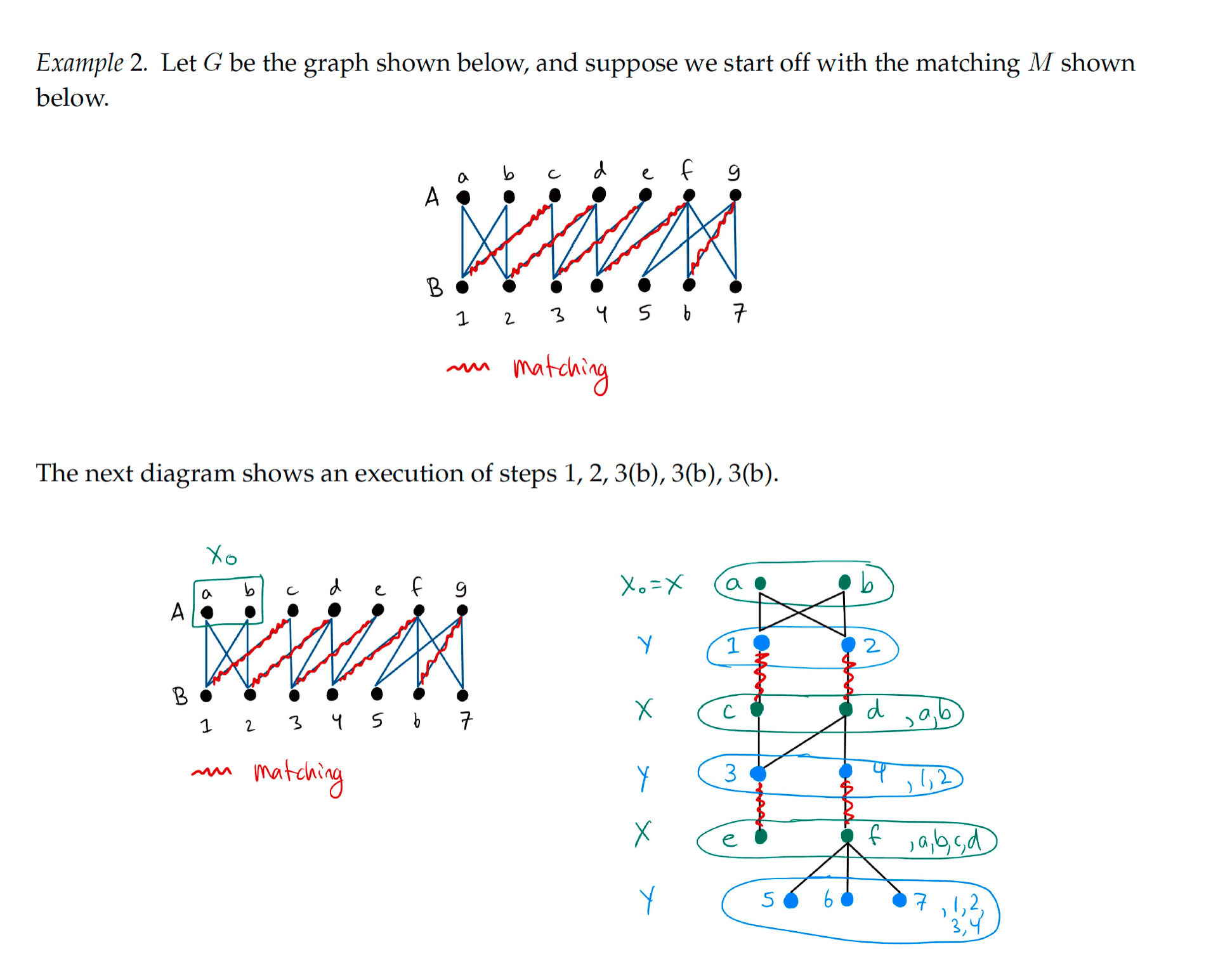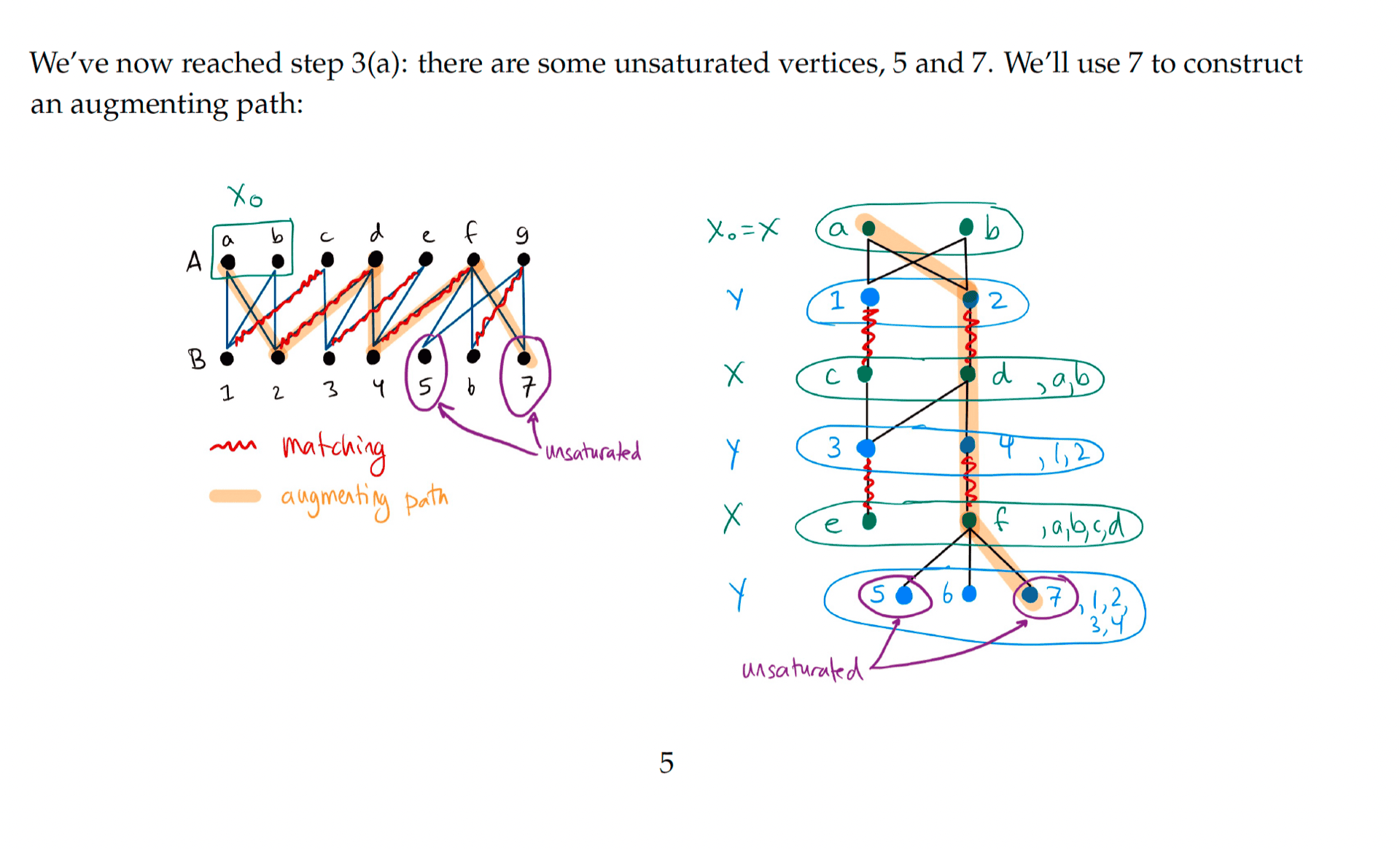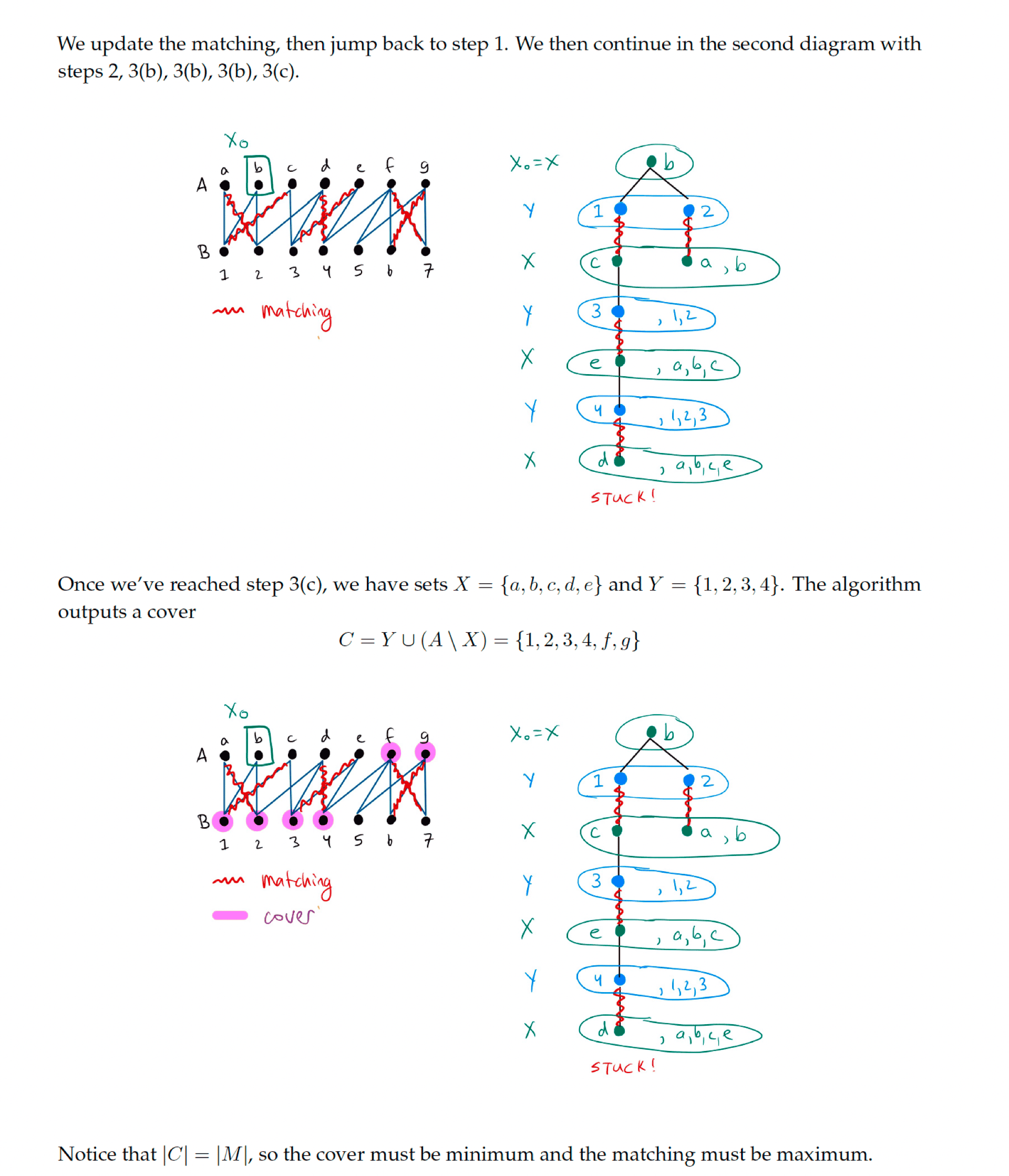# Bipartite Matching Algorithm §

This is an algorithm which looks for augmenting paths, used to prove Konig’s Theorem.

#### Pseudocode §

Given a bipartite graph with bipartition , and a matching of .

1. Let be the set of all unsaturated vertices in .
2. Set and .
3. Find all neighbours of in not currently in .
• (a) If one such vertex is unsaturated, then we have found an augmenting path. Make a larger matching by swapping edges in the augmenting path. Then start over at step 1.
• (b) If all such vertices are saturated, then put all of them in . Add their matching neighbours to . Go to step 3.
• (c) If no such vertices exist, then stop. The matching is maximum, and the minimum cover is .

#### Example §